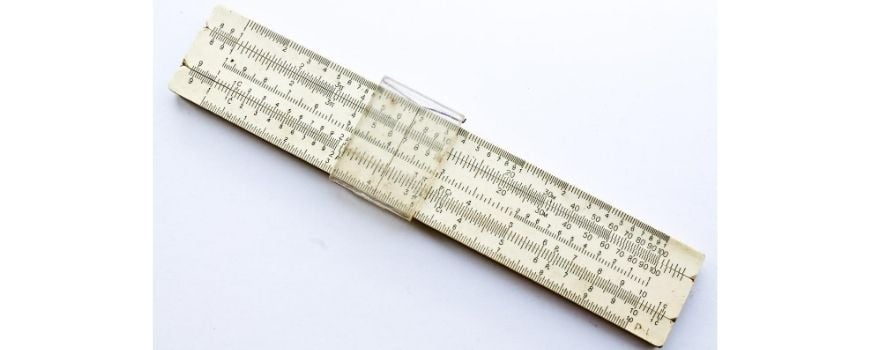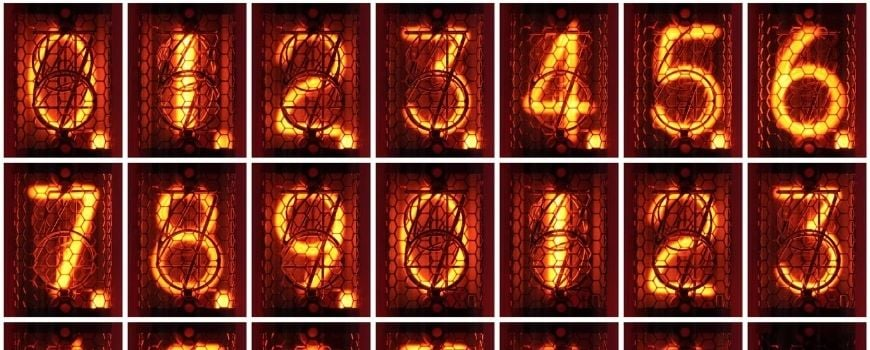# Everything You Need To Know About Significant Figures For Chemistry

Significant figures are a central concept to reporting values in science, but one that is commonly misunderstood. In this article, we will look at the rules behind significant figures and when to apply them.

• Determining significant figures
• Significant figure rules for multiplication and division
• Significant figure rules for addition and subtraction
• Significant figure rules for logs and antilogs
• Summary
• Important note

## Determining significant figures

The number of significant figures of a value can be determined by the following rules:

1. Reading the value from left to right, the first non-zero digit is the first significant figure.
2. If the value has a decimal point, all digits to the right of the first significant figure (zero and non-zero) are significant.

If the value does not have a decimal point, all digits to the right of the first significant figure to the last non-zero digit are significant. Any zeros following this digit are considered trailing zeros and are not significant.

For example,

• $$301.00$$ has 5 significant figures
• $$0.000430$$ has 3 significant figures
• $$5010$$ has 3 significant figures

## Significant figure rules for multiplication and division

For values calculated by multiplication or division, the number of significant figures given in the answer is based on the number of significant figures of the least precise value used in the calculation.

For example,

• $$1.4 \times 607 = 850$$ (2 sig figs)
• $$1.4 \times 600 = 800$$ (1 sig fig)
• $$1.4 \times 600.0 = 840$$ (2 sig figs)
• $$0.40 \times 0.0060 = 0.0024$$ (2 sig figs)

## Significant figure rules for addition and subtraction

For values calculated by addition or subtraction, the answer should contain the same number of decimal places as the least precise value used in the calculation.

For example,

• $$1.2 + 6.50 = 7.7$$ (2 sig figs)
• $$1.2 + 6 = 7$$ (1 sig fig)
• $$0.00540 + 0.00100 = 0.00640$$ (3 sig figs)How well do you know the rules for Logarithms?

## Significant figure rules for logs and antilogs

The significant figure rules for logs and antilogs is a little different. A log value consists of two components: the integer component (the characteristic) and a decimal component (the mantissa).

For example, for the log value $$1.176, \ 1$$ is the characteristic and $$0.176$$ is the mantissa.

The number of significant figures of a log value is based on the mantissa – the characteristic of a log is not considered significant as it only provides information about the order of magnitude of the value.

For example, the log value $$1.176$$ has $$3$$ significant figures.

An antilog is the inverse of a log. The number of significant figures that are given for an antilog value is also based on the decimal component of the log.

Let’s illustrate these ideas with a common usage of logarithms in science: pH values.

$$pH$$ describes the acidity of a solution through the following relationship:

$$pH = -log_{10} [H^{+}]$$, where $$[H^{+}]$$ is the concentration of hydrogen ions (the component of a solution responsible for acidity).

If we have a solution where $$[H^{+}] = 0.100 M$$, the $$pH$$ will be $$1.000$$ (3 sig. figs).

If we have a solution with a $$pH$$ of $$1.00$$, the  $$[H^{+}]$$ will be $$0.10 M$$ (2 sig. figs).This can help remove ambiguity for the number of significant figures of integer values ending in zeros.

For example, $$100$$ could be a value given to $$1, 2 \mbox{or} 3$$ significant figures. Writing $$100$$ (3 sig figs) very clearly shows the number of significant figures of your answer.

Scientific notation can also be used to explicitly show the intended number of significant figures of a value. Returning to our example of $$100$$ , expressing the value as $$1.00 \times 10^{2}$$  clearly shows that the value is to $$3$$ significant figures.

• Do not round off values until the end of your calculation

Rounding values at early stages of your calculations can introduce rounding errors, and ultimately impact your final answer. To reduce the impact of rounding errors, it is a good idea to carry an additional $$2-3$$ extra significant figures in each stage of your calculation until the final answer is given.

• Be mindful of order of operations

When multiple mathematical operations are required, the significant figure rules used are dependent on the order of operations.

For example, let’s consider the following calculation.

\begin{align}
q &= 0.062 \times 0.84 \times 10^{3} \times (80.5 – 25.5) + 0.0050 \times 0.13 \times 10^3 \times (80.5 – 25.5) \\
&= 2900.15 \ J = 2.9 \ kJ \mbox{(2 sig figs)}
\end{align}

## Summary

 Mathematical operation Rule Multiplication and division Answer contains as many significant figures as the least precise value used in the calculation Addition and subtraction Answer contains as many decimal places as the least precise value used in the calculation Logs and antilogs Answer contains as many significant figures as the decimal component of the log value (the mantissa)

## Important note

• These rules are often simplified in schools and the HSC, such that the rule for multiplication and division is applied to all mathematical operations.
• Some schools count all trailing zeros as significant.